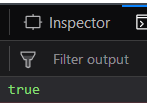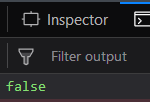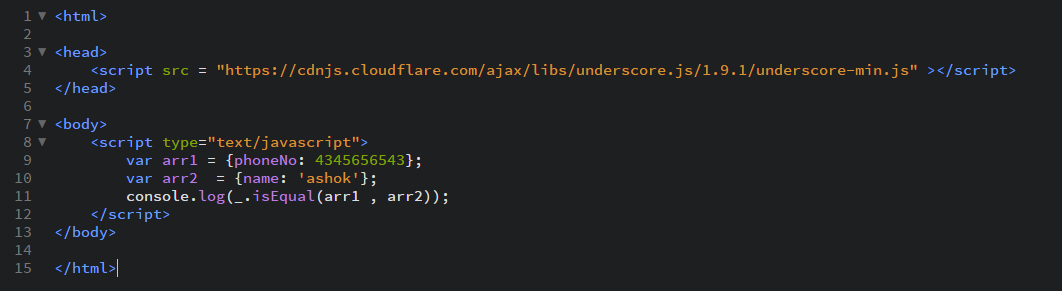# Underscore.js | _.isEqual()

_.isEqual() function:

• It is used to find that whether the 2 given arrays are same or not.
• two arrays are same if they have the same number of elements, both the property and the values need to be same.
• It may be beneficial in situations where the arrays elements are not known and we want to check whether they are same or not.

Syntax:

`_.isEqual(object, other)`

Parameters:
It takes two arguments:

• The object/array
• The other array

Return value:
It returns true if the arrays passed are same otherwise it returns false.

Examples:

1. Passing 2 simple arrays to the _.isEqual() function:
The _.isEqual() function takes the element from the list of one array and searches it in the other array. If that property is found with the same value in the other array then it just goes on to checks the other property otherwise it just returns false. In this it checks for both the character values and the number values in the property.

 `` `<``html``> ` `  `  `<``head``> ` `   ``<``script` `src` `=  ` `    ``"https://cdnjs.cloudflare.com/ajax/libs/underscore.js/1.9.1/underscore-min.js"``> ` `   `` ` `  ` `<``body``> ` `    ``<``script` `type``=``"text/javascript"``> ` `        ``var arr1 = {name: 'akash', numbers: [3, 7, 14]}; ` `        ``var arr2  = {name: 'akash', numbers: [3, 7, 14]}; ` `        ``console.log(_.isEqual(arr1, arr2)); ` `    `` ` ` ` `  `  ` `

Output:2. Passing an array with more properties to the _.isEqual() function:
An array can have as many properties ad it has to act as an parameter to this function. Like here both the arrays contain 3 properties each of type character and date. The _.isEqual() function will work in the same way as for the above example. Since, both the arrays have same properties and the same values so, the output will be ‘true’.

 `` `<``html``> ` `  `  `<``head``> ` `    ``<``script` `src` `=  ` `    ``"https://cdnjs.cloudflare.com/ajax/libs/underscore.js/1.9.1/underscore-min.js"``> ` `    `` ` ` ` `  `  `<``body``> ` `    ``<``script` `type``=``"text/javascript"``> ` `        ``var arr1 = {name: 'akash', gender: ['male'], birthDate: [03/22/99]}; ` `        ``var arr2  = {name: 'akash', gender: ['male'], birthDate: [03/22/99]}; ` `        ``console.log(_.isEqual(arr1, arr2)); ` `    `` ` ` ` `  `  ` `

Output:3. Passing 2 empty arrays to the _.isEqual() function:
The _.isEqual() function will try to check all the array properties along with their values. Since, both the array does not have any property so, there is nothing to match. And hence, both the arrays are equal. Therefore, the answer will be true.

 `<``html``> ` `  `  `<``head``> ` `    ``<``script` `src` `=  ` `    ``"https://cdnjs.cloudflare.com/ajax/libs/underscore.js/1.9.1/underscore-min.js"``> ` `     `` ` ` ` `  `  `<``body``> ` `    ``<``script` `type``=``"text/javascript"``> ` `        ``var arr1 = {}; ` `        ``var arr2  = {}; ` `        ``console.log(_.isEqual(arr1, arr2)); ` `    `` ` ` ` `  `  ` `

Output:4. Passing arrays with different properties to the _.isEqual() function:
If we pass arrays containing different properties then this function will work the same way. It will take the property of first parameter array ( here, ‘name’) and try to find it in the next array. But since, the other array does not have this property so the output will be ‘false’.

 `` ` `  `<``html``> ` `  `  `<``head``> ` `    ``<``script` `src` `=  ` `    ``"https://cdnjs.cloudflare.com/ajax/libs/underscore.js/1.9.1/underscore-min.js"``> ` `    `` ` ` ` `  `  `<``body``> ` `    ``<``script` `type``=``"text/javascript"``> ` `        ``var arr1 = {phoneNo: 4345656543}; ` `        ``var arr2  = {name: 'ashok'}; ` `        ``console.log(_.isEqual(arr1, arr2)); ` `    `` ` ` ` `  `  ` `

Output:5. `

NOTE:
These commands will not work in Google console or in firefox as for these additional files need to be added which they didn’t have added.

 `` `<``script` `type``=``"text/javascript"` `src` `= ` `"https://cdnjs.cloudflare.com/ajax/libs/underscore.js/1.9.1/underscore-min.js"``> ` ` `

An example is shown below:My Personal Notes arrow_drop_upI like to do coding in C++C and java programming languages HTML and CSS always intersts me Sharing knowleged is the best way according to me to increase ones knwoledge

If you like GeeksforGeeks and would like to contribute, you can also write an article using contribute.geeksforgeeks.org or mail your article to contribute@geeksforgeeks.org. See your article appearing on the GeeksforGeeks main page and help other Geeks.

Please Improve this article if you find anything incorrect by clicking on the "Improve Article" button below.

Improved By : shubham_singh